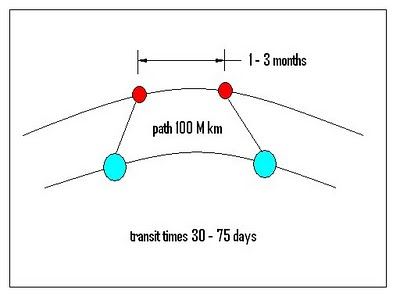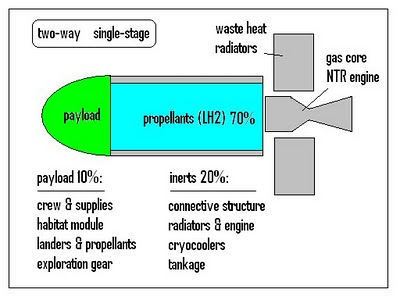## Monday, November 29, 2010

### Fast Transit To and From Mars

Based on some earlier work, I thought I would take a more systematic look at fast trips to and from Mars. I limited this study to gas core nuclear thermal rocket propulsion.

The “typical” close approach distance from Earth to Mars at opposition is some 97 million kilometers. For very fast one-way flight times (on the order of 30 to75 days), the trip is very nearly a straight-line “shot”, at a rather low angle to a radial line. For fairly short stays at Mars (1 to 3 months), this picture doesn’t change much. Accordingly, I chose a nice round figure 100 million km, with an angle factor of 1. I looked at transit times from 30 to 75 days, computing average velocity as path length divided by transit time. A spreadsheet program automated these calculations. See fig.1.Figure 1 – The Fast Transit Orbital Picture

The peak velocity is related to actual vehicle delta-vee. For fairly short impulsive delta-vee events, the velocity versus path length trace is nearly a square wave. The higher the acceleration, the closer the peak velocity is to the average. I assumed for this study the fairly brisk acceleration level of 0.5 gee at departure weight, knowing this would increase by the mass ratio at burnout weight, unless thrust were throttled back by the mass ratio. This takes the form of a manual convergence in the spreadsheet. For a round trip, vehicle delta-vee capability is 4 times this peak velocity.

Assumed Vehicle Characteristics:

The vehicle assumed for this generic trade study is depicted in the fig. 2 cartoon. This vehicle is single stage, 10% payload, 20% inerts, and 70% propellant. This corresponds to a vehicle mass ratio of 3-1/3.

Payload includes the crew, their habitat and supplies, their crew return vehicles, the Mars landing craft plus their propellants and all the exploration gear. All of this landing and exploration equipment is assumed to be returned to Earth, which is not realistic, but is very conservative. That “covers” the lack of a propellant “margin” in these figures.

Inerts include the vehicle connective structures, auxiliary electric power generating equipment, tank structures and insulation, cryo-cooler equipment to keep the liquid hydrogen liquid for months at a time, the basic gas core fission engine, and the waste heat radiator equipment which allows the engine to operate at Isp greater than about 2000 sec. In particular, the radiator could be a very major portion of that 20% inerts figure. It is unknown whether this is really adequate.

The propellant figure is the liquid hydrogen working fluid for the gas core fission nuclear thermal rocket engine.Figure 2 – Basic Vehicle Assumptions

Velocity Requirements:

From the velocity trace modeled in the spreadsheet, we calculate average velocity versus one-way travel time, and peak velocity from the average using a constant 0.5 gee acceleration level. These are plotted in fig.3. At this level of acceleration, average and peak velocities are virtually indistinguishable. Required vehicle delta-vee for a one-way trip is twice the peak velocity, four times peak for a two-way trip, as plotted in fig. 4Figure 3 – Average and Peak Transit VelocitiesFigure 4 – Estimated Vehicle Delta-Vee Requirements

Assumed Propulsion Characteristics:

The focus here is on one type of propulsion: open-cycle gas core nuclear thermal rocketry. This is something that came very close to propulsive test in the late 1960’s.

At the end of the 60’s, controlled gas-phase fission had been demonstrated, as well as a powered-plasma flow model simulating a hydrogen to uranium flow ratio of 1000:1, which was as good as perfect containment. It was known that a waste heat radiator would be required, but not how it could be built, nor how much it would really weigh.

Neither the characteristics of that radiator, nor the maximum impulse capability of such systems, were known at that time. However, a first generation design concept was targeted at specific impulse Isp = 6000 seconds, because they were pretty sure they could achieve it. That is the screening criterion used in this study.

Estimated Propulsive Requirements:

From the mass ratio and the two-way trip delta-vee figures, the exhaust velocity required of the engine can be estimated. Dividing this by the gravity constant is a rather good estimate of the engine Isp required.

From the previous study, the payload for a crew of 6,twolanders,and two crew return capsules was 240 metric tons. Using this figure and the 10% payload fraction provides a constant estimate of Earth orbit departure weight, shown in fig.5 with the Isp.Figure 5 – Required Isp and Departure Weight

The acceleration gee level at departure was assumed to be 0.5 for this study. At burnout weight on return, the acceleration is higher by the mass ratio, assuming constant thrust level. Under the constant thrust level assumption, the departure weight and acceleration set the engine thrust level. For these figures, the departure weight is 2400 metric tons, so the thrust level is 1200 metric “tons-force”, or 1,200,000 “kg-force”, which is some 11.76 mega-Newtons of force in proper SI units.

Thrust divided by Isp is propellant flow rate. Propellant mass divided by flow rate is the total burn time available to “dry tanks”. This was converted to hours for easy plotting. Vehicle departure and burnout gees, plus total burn time to dry tanks, is given in fig. 6.Figure 6 – Acceleration and Burn Time Data

Conclusions:
This oversimplified study is for a single-stage two-way trip: everything goes and returns, no expendables. From the standpoint of Isp’s thought feasible, it looks like such a vehicle could be powered by a gas core fission rocket, if the one-way travel time were no shorter than about 65 days. This is for an “average” opposition mission.

Possible major refinements: (1) reduce lander mass in favor of more lander propellants (extra landings) by taking some advantage of descent aerobraking, and (2) leave landers, unused lander propellants, and some small portion of the vehicle tankage behind in Mars orbit, as a depot for subsequent missions, thereby reducing payload and inerts for the return home.